Open In App

# Ratio of area of two nested polygons formed by connecting midpoints of sides of a regular N-sided polygon

Given an N-sided polygon, the task is to find the ratio of the area of the Nth to (N + 1)th N-sided regular nested polygons generated by joining the midpoints of the sides of the original polygon.

Examples :

Input: N = 3
Output: 4.000000
Explanation: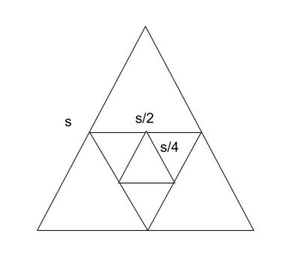Nested Triangle

Ratio of the length of the sides formed by joining the mid-points of the triangle with the length of the side of the original triangle is 0.5. Hence, R = (Area of Nth triangle) / (Area of (N + 1)th triangle) = 4

Input: N = 4
Output: 2.000000

Approach: The problem can be solved based on the following observations:

• Consider an N-sided regular polygon as shown in the figure below.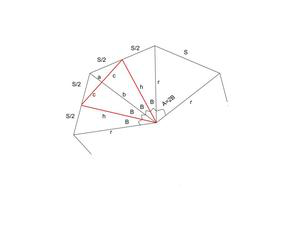Representation Of Nested regular polygon of N sides.

• A = 2 * ? / N
B = ? / N
h = r * cos(B)
b = h * cos(B)
c = h((1 – cos(A)) / 2)1/2
• Area of the Black Isosceles Triangle: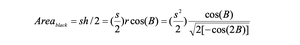• Area of the Red Isosceles Triangle: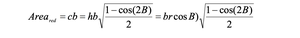• r = s / (2 * [1 – cos(2B)])1/2 and b = r * [cos(B)]2
• After combining the above equations: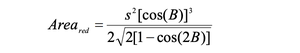• Final result obtained is as follows: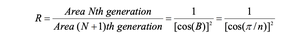Below is the implementation of the above approach:

## C++

 `// C++ code for the above approach``#include ``using` `namespace` `std;` `// Function to calculate the ratio of``// area of N-th and (N + 1)-th nested``// polygons formed by connecting midpoints``void` `AreaFactor(``int` `n)``{``    ``// Stores the value of PI``    ``double` `pi = 3.14159265;` `    ``// Calculating area the factor``    ``double` `areaf = 1 / (``cos``(pi / n)``                        ``* ``cos``(pi / n));` `    ``// Printing the ratio``    ``// precise upto 6 decimal places``    ``cout << fixed << setprecision(6)``         ``<< areaf << endl;``}` `// Driver Code``int` `main()``{``    ``int` `n = 4;``    ``AreaFactor(n);` `    ``return` `0;``}`

## Java

 `// Java program for the above approach``import` `java.io.*;` `class` `GFG{` `// Function to calculate the ratio of``// area of N-th and (N + 1)-th nested``// polygons formed by connecting midpoints``static` `void` `AreaFactor(``int` `n)``{``    ` `    ``// Stores the value of PI``    ``double` `pi = ``3.14159265``;`` ` `    ``// Calculating area the factor``    ``double` `areaf = ``1` `/ (Math.cos(pi / n) *``                        ``Math.cos(pi / n));`` ` `    ``// Printing the ratio``    ``// precise upto 6 decimal places``    ``System.out.format(``"%.6f"``, areaf);``}` `// Driver Code``public` `static` `void` `main(String[] args)``{``    ``int` `n = ``4``;``    ` `    ``AreaFactor(n);``}``}` `// This code is contributed by code_hunt`

## Python3

 `# Python3 code for the above approach``import` `math` `# Function to calculate the ratio of``# area of N-th and (N + 1)-th nested``# polygons formed by connecting midpoints``def` `AreaFactor(n):` `    ``# Stores the value of PI``    ``pi ``=` `3.14159265` `    ``# Calculating area the factor``    ``areaf ``=` `1` `/` `(math.cos(pi ``/` `n) ``*``                 ``math.cos(pi ``/` `n))` `    ``# Printing the ratio``    ``# precise upto 6 decimal places``    ``print``(``'%.6f'` `%` `areaf)``    ` `# Driver Code``if` `__name__ ``=``=` `"__main__"``:``  ` `    ``n ``=` `4``    ``AreaFactor(n)` `# This code is contributed by ukasp`

## C#

 `// C# program for the above approach``using` `System;``using` `System.Collections.Generic;` `class` `GFG {` `// Function to calculate the ratio of``// area of N-th and (N + 1)-th nested``// polygons formed by connecting midpoints``static` `void` `AreaFactor(``int` `n)``{``     ` `    ``// Stores the value of PI``    ``double` `pi = 3.14159265;``  ` `    ``// Calculating area the factor``    ``double` `areaf = 1 / (Math.Cos(pi / n) *``                        ``Math.Cos(pi / n));``  ` `    ``// Printing the ratio``    ``// precise upto 6 decimal places``    ``Console.WriteLine(Math.Round(areaf));` `}``    ``// Driver Code``    ``public` `static` `void` `Main(``string``[] args)``    ``{``        ``int` `n = 4;``     ` `        ``AreaFactor(n);``    ``}``}` `// This code is contributed by susmitakundugoaldanga.`

## Javascript

 ``

Output:

`2.000000`

Time Complexity: O(1)
Auxiliary Space: O(1)